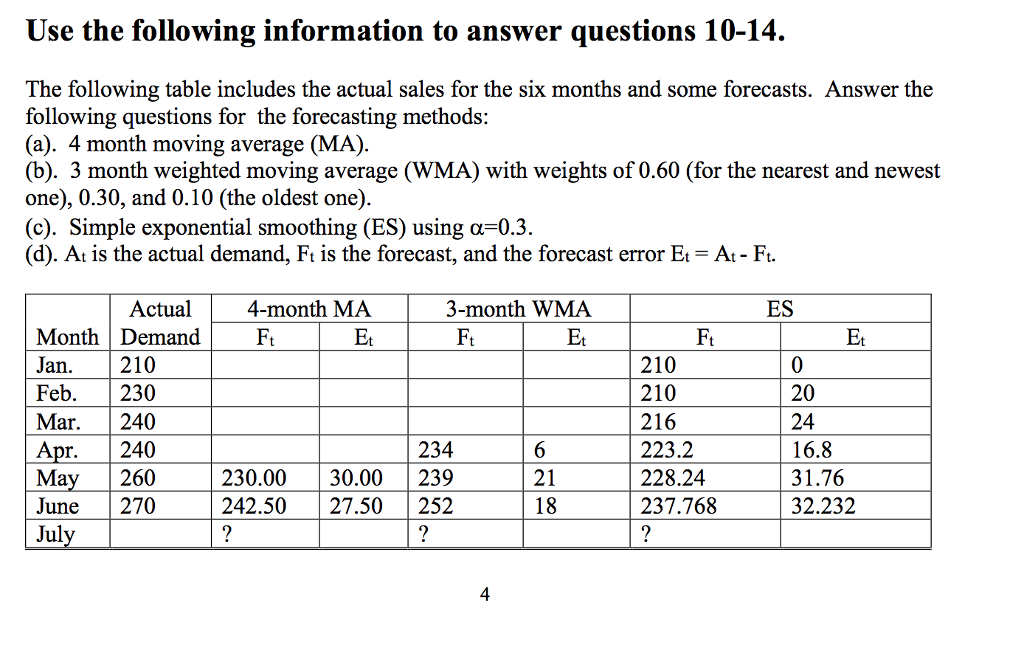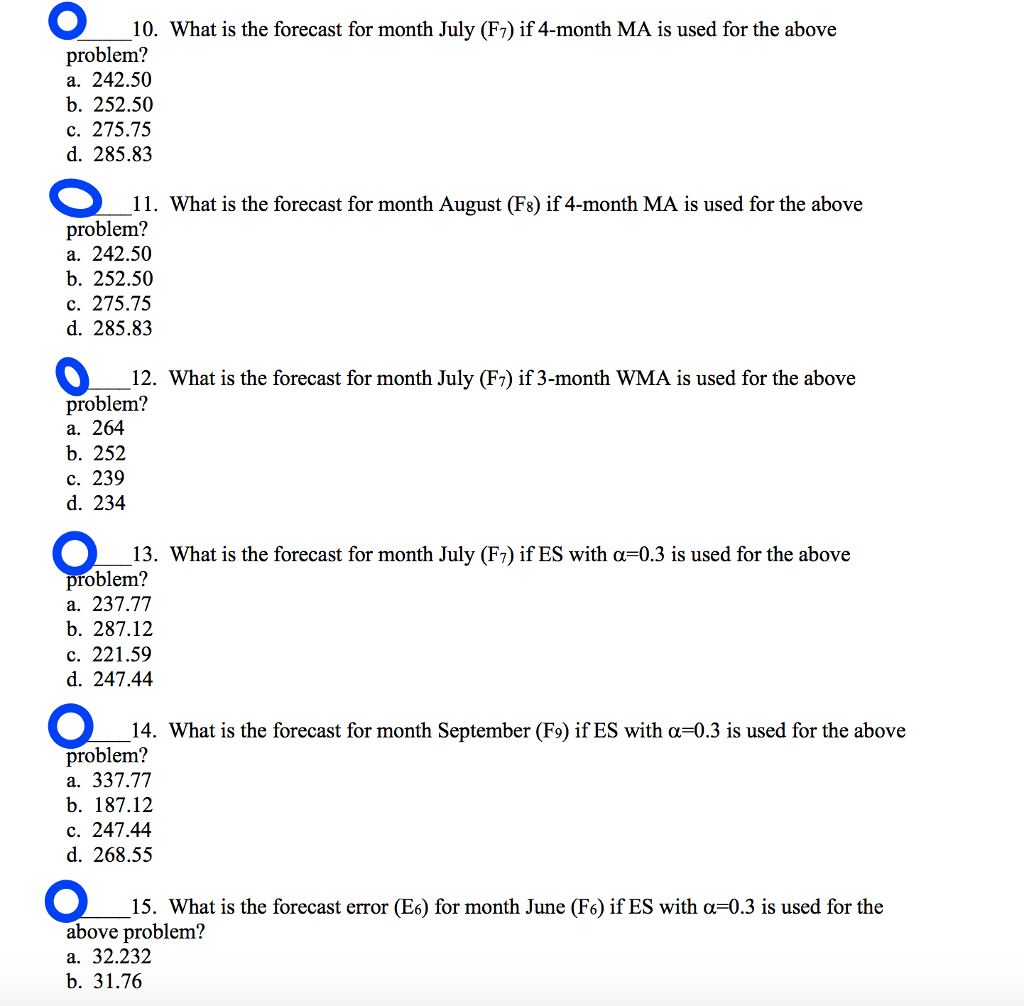# Answered! Hi Folks, I am really getting confused here, I dont know how to solve these forcasting problems. I would really…

Hi Folks, I am really getting confused here, I dont know how to solve these forcasting problems. I would really apprecite it. Please please show me the work and how you came to the answers? I dont need directly answers without showing me the calcualtions and the method how you got to those answers please. I want to learn and understand the materials.10.

Formula for forecast using Four month moving average = sum of actual demands of previous four months / 4

Don't use plagiarized sources. Get Your Custom Essay on
Answered! Hi Folks, I am really getting confused here, I dont know how to solve these forcasting problems. I would really…
GET AN ESSAY WRITTEN FOR YOU FROM AS LOW AS \$13/PAGE

So the forecast for July = ( March demand+ April demand+ May demand + June Demand) / 4

= (240+240+260+270)/4 = 252.5

11.

As the actual demand of July is not known to calculate the forecast of August, we need to assume the forecast of July is same as forecast of August.

So the forecast of August = 252.5

12.

Weights for nearest , newest and oldest month are 0.6, 0.3 and 0.1

As per the 3 month weighted moving average method , the forecast for the july month= 0.6 * June month actual demand + 0.3*may month actual Demand+ 0.10* April month actual demand= 0.60*270+0.30*260+0.1*240= 264

13. alphs (@ ) = 0.30

As per exponential smoothing formula ,

forecast for a month = @ * previous month demand + ( 1-@) * previous month exponental ( ES) forecast

Forecast for July = 0.3 * June month demand + ( 1-0.30 )* June month forecast = 0.30 * 270 + ( 1-0.3) * 237.768= 247.44

14. Forecast error for june = Actual demand of June – Exponential forecast for June = 270-237.68= 32.232

As forecast and actual demand of August ( which is previous month of September) is not known. So Forecasst of spetember cannot be calculated.

So we need to assume forecast of july is same as forecast for August and September = 247.44